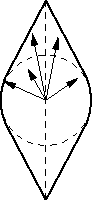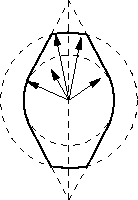Next: Geomorphing Up: Implementation Previous: Implementation

## Deviation spaces

A deviation space as used in  is shown in figure

 [HOPPE ()][extended]4(a). The error vectors (representing the geometric deviation between the original and the simplified mesh) are bounded by a volume consisting of a sphere and two cones. In this implementation the deviation spaces are ``clipped'' at a sphere large enough to contain all error vectors (figure 4(b)). Thus the tops of the cones are cut off resulting in less detail drawn without exceeding the screen-space error tolerance.

After a vertex vs is created by an edge collapse operation, for all leaf nodes of the hierarchy tree below vs (vertices of the original mesh) their nearest neighbor on Nvs (all faces adjacent to vs) is computed. The error vectors are the deviations between a leaf vertex and its nearest neighbor on Nvs. By descending the PM hierarchy it is also guaranteed that only topological corresponding regions are considered (as in ).

Markus Grabner